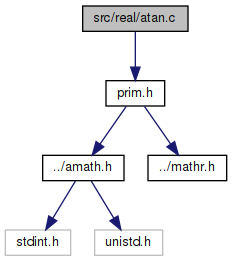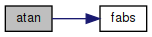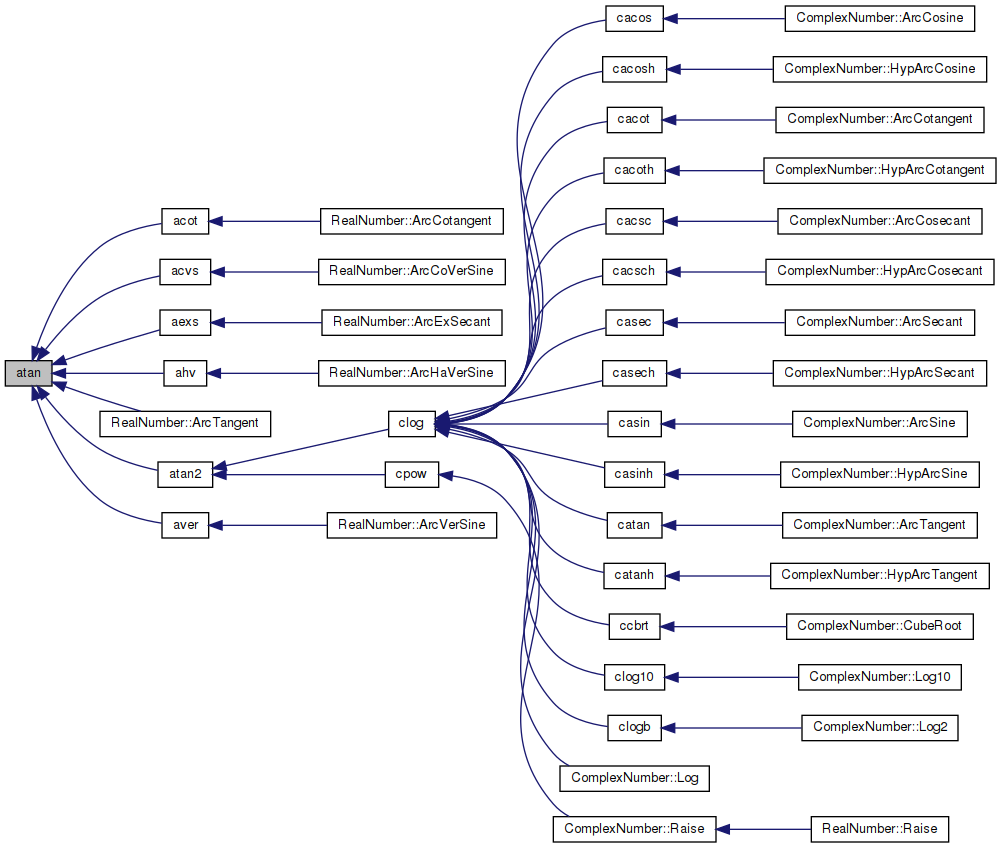amath  1.8.5 Simple command line calculator
atan.c File Reference

Inverse tangent function. More...

`#include "prim.h"`
Include dependency graph for atan.c:Go to the source code of this file.

## Functions

double atan (double x)
Inverse tangent function. More...

## Variables

static const double atanhi []

static const double atanlo []

static const double aT []

static const double one = 1.0

static const double huge = 1.0e300

## Detailed Description

Inverse tangent function.

Definition in file atan.c.

## ◆ atan()

 double atan ( double x )

Inverse tangent function.

```Method
1. Reduce x to positive by atan(x) = -atan(-x).
2. According to the integer k=4t+0.25 chopped, t=x, the argument
is further reduced to one of the following intervals and the
arctangent of t is evaluated by the corresponding formula:```
```    [0,7/16]      atan(x) = t-t^3*(a1+t^2*(a2+...(a10+t^2*a11)...)
[7/16,11/16]  atan(x) = atan(1/2) + atan( (t-0.5)/(1+t/2) )
[11/16.19/16] atan(x) = atan( 1 ) + atan( (t-1)/(1+t) )
[19/16,39/16] atan(x) = atan(3/2) + atan( (t-1.5)/(1+1.5t) )
[39/16,INF]   atan(x) = atan(INF) + atan( -1/t )```
```Constants
The hexadecimal values are the intended ones for the following
constants. The decimal values may be used, provided that the
compiler will convert from decimal to binary accurately enough
to produce the hexadecimal values shown.
```

Definition at line 103 of file atan.c.

References aT, atanhi, atanlo, fabs(), huge, and one.

Referenced by acot(), acvs(), aexs(), ahv(), RealNumber::ArcTangent(), atan2(), and aver().

104 {
105  double w, s1, s2, z;
106  int32_t ix, hx, id;
107
108  GET_HIGH_WORD(hx, x);
109  ix = hx & 0x7fffffff;
110  if (ix >= 0x44100000)
111  { /* if |x| >= 2^66 */
112  uint32_t low;
113
114  GET_LOW_WORD(low, x);
115  if (ix > 0x7ff00000 || (ix == 0x7ff00000 && (low != 0)))
116  return NAN;
117  if (hx > 0)
118  return atanhi + atanlo;
119  else
120  return -atanhi - atanlo;
121  }
122  if (ix < 0x3fdc0000)
123  { /* |x| < 0.4375 */
124  if (ix < 0x3e200000)
125  { /* |x| < 2^-29 */
126  if (huge + x > one)
127  return x; /* raise inexact */
128  }
129  id = -1;
130  }
131  else
132  {
133  x = fabs(x);
134  if (ix < 0x3ff30000)
135  { /* |x| < 1.1875 */
136  if (ix < 0x3fe60000)
137  { /* 7/16 <=|x|<11/16 */
138  id = 0;
139  x = (2.0 * x - one) / (2.0 + x);
140  }
141  else
142  { /* 11/16<=|x|< 19/16 */
143  id = 1;
144  x = (x - one) / (x + one);
145  }
146  }
147  else
148  {
149  if (ix < 0x40038000)
150  { /* |x| < 2.4375 */
151  id = 2;
152  x = (x - 1.5) / (one + 1.5 * x);
153  }
154  else
155  { /* 2.4375 <= |x| < 2^66 */
156  id = 3;
157  x = -1.0 / x;
158  }
159  }
160  }
161  /* end of argument reduction */
162  z = x * x;
163  w = z * z;
164  /* break sum from i=0 to 10 aT[i]z**(i+1) into odd and even poly */
165  s1 = z * (aT + w * (aT + w * (aT + w * (aT + w * (aT + w * aT)))));
166  s2 = w * (aT + w * (aT + w * (aT + w * (aT + w * aT))));
167  if (id < 0)
168  {
169  return x - x * (s1 + s2);
170  }
171
172  z = atanhi[id] - ((x * (s1 + s2) - atanlo[id]) - x);
173  return (hx < 0) ? -z : z;
174 }
#define GET_HIGH_WORD(i, d)
Get the more significant 32 bit int from a double.
Definition: prim.h:167
static const double huge
Definition: atan.c:78
#define GET_LOW_WORD(i, d)
Get the less significant 32 bit int from a double.
Definition: prim.h:177
static const double aT[]
Definition: atan.c:62
static const double atanhi[]
Definition: atan.c:48
#define NAN
Definition: mathr.h:53
static const double one
Definition: atan.c:77
static const double atanlo[]
Definition: atan.c:55
double fabs(double x)
Returns the absolute value of x.
Definition: fabs.c:51
Here is the call graph for this function:Here is the caller graph for this function:## ◆ aT

 const double aT[]
static
Initial value:
= {
3.33333333333329318027e-01,
-1.99999999998764832476e-01,
1.42857142725034663711e-01,
-1.11111104054623557880e-01,
9.09088713343650656196e-02,
-7.69187620504482999495e-02,
6.66107313738753120669e-02,
-5.83357013379057348645e-02,
4.97687799461593236017e-02,
-3.65315727442169155270e-02,
1.62858201153657823623e-02,
}

Definition at line 62 of file atan.c.

Referenced by atan().

## ◆ atanhi

 const double atanhi[]
static
Initial value:
= {
4.63647609000806093515e-01,
7.85398163397448278999e-01,
9.82793723247329054082e-01,
1.57079632679489655800e+00,
}

Definition at line 48 of file atan.c.

Referenced by atan().

## ◆ atanlo

 const double atanlo[]
static
Initial value:
= {
2.26987774529616870924e-17,
3.06161699786838301793e-17,
1.39033110312309984516e-17,
6.12323399573676603587e-17,
}

Definition at line 55 of file atan.c.

Referenced by atan().

## ◆ huge

 const double huge = 1.0e300
static

Definition at line 78 of file atan.c.

Referenced by atan().

## ◆ one

 const double one = 1.0
static

Definition at line 77 of file atan.c.

Referenced by atan().